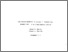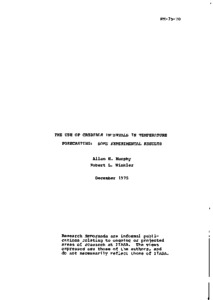# The Use of Credible Intervals in Temperature Forecasting: Some Experimental Results

Murphy, A.H. & Winkler, R.L. (1975). The Use of Credible Intervals in Temperature Forecasting: Some Experimental Results. IIASA Research Memorandum. IIASA, Laxenburg, Austria: RM-75-070Preview Text RM-75-070.pdf Download (582kB) | Preview

## Abstract

Probability can be thought of as the language of uncertainty, and, as such, it provides forecasters with a means of describing the uncertainty inherent in their forecasts in a formal, quantitative manner. Probability forecasts, in turn, provide potential users of forecasts with information required to make rational decisions in uncertain situations.

Since 1965, the National Weather Service (NWS) in the United States has routinely issued precipitation probability forecasts to the general public. Forecasts of maximum and minimum temperature, however, are still expressed in categorical terms (i.e., in terms of a specific temperature or a range of temperatures). In this paper we describe and compare the results of two recent experiments in which NWS forecasters used credible intervals to describe the uncertainty inherent in their temperature forecasts. A credible interval temperature forecast is simply an interval forecast accompanied by the forecaster's subjective probability that the temperature of concern will fall in the interval.

The experiments were conducted in the NWS forecast offices in Denver, Colorado and Milwaukee, Wisconsin and involved four and five forecasters, respectively. In each experiment, one group of forecasters made variable-width interval forecasts and the other group made fixed-width interval forecasts. In the variable-width approach, the forecasters determined 50% and 75% central credible intervals using the "method of successive sub-divisions" (i.e., they assessed the median, 25th percentile, 12.5th percentile, 75th percentile, 87.5th percentile of their probability distributions, in that order). In the fixed-width approach, the forecasters first determined a median and then assessed probabilities for 5 degrees F and 9 degrees F intervals centered at the median.

In evaluating the results of the experiments, several properties of the forecasts (i.e., the medians and the intervals) were of interest, including their reliability and precision. Reliability refers to the degree of correspondence between the probabilities associated with the forecasts and the sample relative frequencies (i.e., the distribution of observed temperatures), while precision relates to the degree of correspondence between the forecasts and the observed temperatures on an individual basis. The latter can be measured by computing the average absolute error (and similar quantities) in the case of the medians and average scores based upon one or more "proper" scoring rules in the case of the (fixed-width) intervals. The forecasts formulated by the forecasters were also compared with forecasts based solely upon climatological data. The results of the experiments indicate that NWS forecasters can formulate reliable and precise credible interval temperature forecasts and that these forecasts are generally better (in the sense of these two properties) than forecasts based upon climatological data.

The influences of a number of variables were considered in the process of evaluating the forecasts. These variables included: 1) location of experiment (Denver, Milwaukee); 2) type of interval (variable-width, fixed-width); 3) type of temperature (maximum, minimum); 4) forecast length (12 hours, 24 hours, 36 hours) ; and 5) forecaster. In addition, factors such as forecasting experience, training, and learning effects were investigated within the constraints imposed by the number of forecasters and the length of the experiments.

Finally, we briefly discuss the implications of these experiments and the results for probability forecasting in general and probability forecasting in meteorology in particular.

Item Type: Monograph (IIASA Research Memorandum) Energy Program (ENP) IIASA Import 15 Jan 2016 01:42 27 Aug 2021 17:08 https://pure.iiasa.ac.at/446View Item